# Teach you to design template class library to get K-line data of specified length

Author: Lydia, Created: 2023-06-29 17:27:59, Updated: 2023-09-18 19:33:33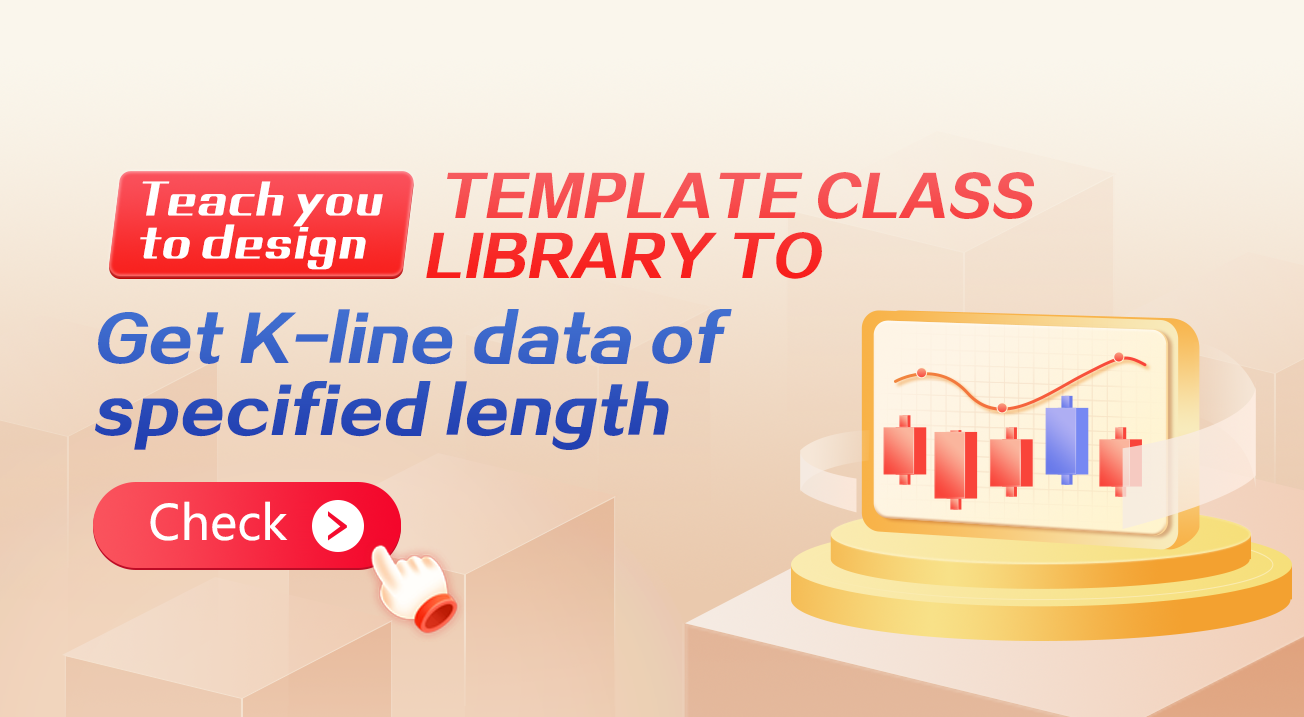# Teach you to design template class library to get K-line data of specified length

When designing trend strategies, it is often necessary to have a sufficient number of K-line bars for calculating indicators. The calculation of indicators relies on the data provided by the `exchange.GetRecords()` function in the FMZ platform API, which is a wrapper for the exchange’s K-line interface. In the early design of cryptocurrency exchange APIs, there was no support for pagination in the K-line interface, and the exchange’s K-line interface only provided a limited amount of data. As a result, some developers were unable to meet the requirements for calculating indicators with larger parameter values.

The K-line interface of Binance’s contract API supports pagination. In this article, we will use the Binance K-line API interface as an example to teach you how to implement pagination and specify the number of bars to retrieve using the FMZ platform template library.

## K-line interface of Binance

K-line data

The opening time of each K-line in the `GET /dapi/v1/klines` endpoint can be considered as a unique ID.

The weight of the request depends on the value of the “LIMIT” parameter.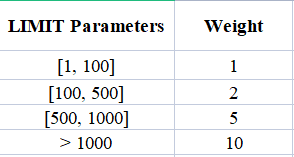Parameters: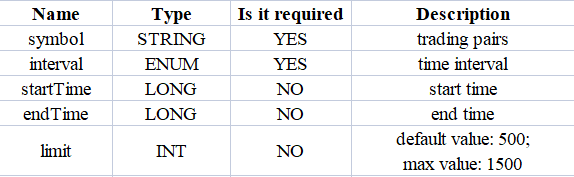First, we need to refer to the exchange’s API documentation to understand the specific parameters of the K-line interface. We can see that when calling this K-line endpoint, we need to specify the type, the K-line period, the data range (start and end time), and the number of pages, etc.

Since our design requirement is to query a specific number of K-line data, for example, to query the 1-hour K-line, 5000 bars of 1-hour K-line data from the current moment towards the past, it is evident that making a single API call to the exchange will not retrieve the desired data.

To achieve this, we can implement pagination and divide the query into segments from the current moment towards a specific historical moment. Since we know the desired K-line data’s period, we can easily calculate the start and end time for each segment. We can then query each segment in sequence towards the historical moment until we retrieve enough bars. The approach sounds simple, so let’s go ahead and implement it!

## Design “JavaScript version of paginated query K-line historical data template”

Interface function for design templates: `\$.GetRecordsByLength(e, period, length)`.

``````/**
* desc: \$.GetRecordsByLength is the interface function of this template library, this function is used to get the K-line data of the specified K-line length
* @param {Object} e - exchange object
* @param {Int} period - K-line period, in seconds
* @param {Int} length - Specify the length of the acquired K-line data, which is related to the exchange interface limits
* @returns {Array<Object>} - K-line data
*/
``````

Design the function `\$.GetRecordsByLength`, which is typically used in the initial stage of strategy execution to calculate indicators based on a long period of K-line data. Once this function is executed and sufficient data is obtained, only new K-line data needs to be updated. There is no need to call this function again to retrieve excessively long K-line data, as it would result in unnecessary API calls.

Therefore, it is also necessary to design an interface for subsequent data updates: `\$.UpdataRecords(e, records, period)`.

``````/**
* desc: \$.UpdataRecords is the interface function of this template library, this function is used to update the K-line data.
* @param {Object} e - exchange object
* @param {Array<Object>} records - K-line data sources that need to be updated
* @param {Int} period - K-line period, needs to be the same as the K-line data period passed in the records parameter
* @returns {Bool}  - Whether the update was successful
*/
``````

The next step is to implement these interface functions.

``````/**
* desc: \$.GetRecordsByLength is the interface function of this template library, this function is used to get the K-line data of the specified K-line length
* @param {Object} e - exchange object
* @param {Int} period - K-line period, in seconds
* @param {Int} length - Specify the length of the acquired K-line data, which is related to the exchange interface limits
* @returns {Array<Object>} - K-line data
*/
\$.GetRecordsByLength = function(e, period, length) {
if (!Number.isInteger(period) || !Number.isInteger(length)) {
throw "params error!"
}

var exchangeName = e.GetName()
if (exchangeName == "Futures_Binance") {
return getRecordsForFuturesBinance(e, period, length)
} else {
throw "not support!"
}
}

/**
* desc: getRecordsForFuturesBinance, the specific implementation of the function to get K-line data for Binance Futures Exchange
* @param {Object} e - exchange object
* @param {Int} period - K-line period, in seconds
* @param {Int} length - Specify the length of the acquired K-line data, which is related to the exchange interface limits
* @returns {Array<Object>} - K-line data
*/
function getRecordsForFuturesBinance(e, period, length) {
var contractType = e.GetContractType()
var currency = e.GetCurrency()
var strPeriod = String(period)

var symbols = currency.split("_")
var baseCurrency = ""
var quoteCurrency = ""
if (symbols.length == 2) {
baseCurrency = symbols
quoteCurrency = symbols
} else {
throw "currency error!"
}

var realCt = e.SetContractType(contractType)["instrument"]
if (!realCt) {
throw "realCt error"
}

// m -> minute; h -> hour; d -> day; w -> week; M -> month
var periodMap = {}
periodMap[(60).toString()] = "1m"
periodMap[(60 * 3).toString()] = "3m"
periodMap[(60 * 5).toString()] = "5m"
periodMap[(60 * 15).toString()] = "15m"
periodMap[(60 * 30).toString()] = "30m"
periodMap[(60 * 60).toString()] = "1h"
periodMap[(60 * 60 * 2).toString()] = "2h"
periodMap[(60 * 60 * 4).toString()] = "4h"
periodMap[(60 * 60 * 6).toString()] = "6h"
periodMap[(60 * 60 * 8).toString()] = "8h"
periodMap[(60 * 60 * 12).toString()] = "12h"
periodMap[(60 * 60 * 24).toString()] = "1d"
periodMap[(60 * 60 * 24 * 3).toString()] = "3d"
periodMap[(60 * 60 * 24 * 7).toString()] = "1w"
periodMap[(60 * 60 * 24 * 30).toString()] = "1M"

var records = []
var url = ""
if (quoteCurrency == "USDT") {
// GET https://fapi.binance.com  /fapi/v1/klines  symbol , interval , startTime , endTime , limit
// limit maximum value:1500

url = "https://fapi.binance.com/fapi/v1/klines"
} else if (quoteCurrency == "USD") {
// GET https://dapi.binance.com  /dapi/v1/klines  symbol , interval , startTime , endTime , limit
// The difference between startTime and endTime can be up to 200 days.
// limit maximum value:1500

url = "https://dapi.binance.com/dapi/v1/klines"
} else {
throw "not support!"
}

var maxLimit = 1500
var interval = periodMap[strPeriod]
if (typeof(interval) !== "string") {
throw "period error!"
}

var symbol = realCt
var currentTS = new Date().getTime()

while (true) {
// Calculate limit
var limit = Math.min(maxLimit, length - records.length)
var barPeriodMillis = period * 1000
var rangeMillis = barPeriodMillis * limit
var twoHundredDaysMillis = 200 * 60 * 60 * 24 * 1000

if (rangeMillis > twoHundredDaysMillis) {
limit = Math.floor(twoHundredDaysMillis / barPeriodMillis)
rangeMillis = barPeriodMillis * limit
}

var query = `symbol=\${symbol}&interval=\${interval}&endTime=\${currentTS}&limit=\${limit}`
var retHttpQuery = HttpQuery(url + "?" + query)

var ret = null
try {
ret = JSON.parse(retHttpQuery)
} catch(e) {
Log(e)
}

if (!ret || !Array.isArray(ret)) {
return null
}

// When the data cannot be searched because it is beyond the searchable range of the exchange
if (ret.length == 0 || currentTS <= 0) {
break
}

for (var i = ret.length - 1; i >= 0; i--) {
var ele = ret[i]
var bar = {
Time : parseInt(ele),
Open : parseFloat(ele),
High : parseFloat(ele),
Low : parseFloat(ele),
Close : parseFloat(ele),
Volume : parseFloat(ele)
}

records.unshift(bar)
}

if (records.length >= length) {
break
}

currentTS -= rangeMillis
Sleep(1000)
}

return records
}

/**
* desc: \$.UpdataRecords is the interface function of this template library, this function is used to update the K-line data.
* @param {Object} e - exchange object
* @param {Array<Object>} records - K-line data sources that need to be updated
* @param {Int} period - K-line period, needs to be the same as the K-line data period passed in the records parameter
* @returns {Bool}  - Whether the update was successful
*/
\$.UpdataRecords = function(e, records, period) {
var r = e.GetRecords(period)
if (!r) {
return false
}

for (var i = 0; i < r.length; i++) {
if (r[i].Time > records[records.length - 1].Time) {
records.push(r[i])
// Update the previous Bar
if (records.length - 2 >= 0 && i - 1 >= 0 && records[records.length - 2].Time == r[i - 1].Time) {
records[records.length - 2] = r[i - 1]
}
} else if (r[i].Time == records[records.length - 1].Time) {
// Update Bar
records[records.length - 1] = r[i]
}
}
return true
}
``````

In the template, we have only implemented support for the Binance futures contract K-line interface, i.e., the `getRecordsForFuturesBinance` function. It can also be extended to support K-line interfaces of other cryptocurrency exchanges.

## Test Session

As you can see, the code for implementing these functionalities in the template is not extensive, totaling less than 200 lines. After writing the template code, testing is crucial and should not be overlooked. Moreover, for data retrieval like this, it is important to conduct thorough testing.

To test it, you need to copy both the “JavaScript Version of Pagination Query K-Line Historical Data Template” and the “Plot Library” templates to your strategy library (which can be found in the Strategy Square ). Then, create a new strategy and select these two templates.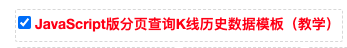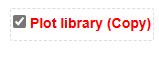The “Plot Library” is used, because we need to draw the obtained K-line data for observation.

``````function main() {
LogReset(1)
var testPeriod = PERIOD_M5
Log("Current exchanges tested:", exchange.GetName())

// If futures, you need to set up a contract
exchange.SetContractType("swap")

// Get K-line data of specified length using \$.GetRecordsByLength
var r = \$.GetRecordsByLength(exchange, testPeriod, 8000)
Log(r)

// Use the Plot test for easy observation
\$.PlotRecords(r, "k")

// Test data
var diffTime = r.Time - r.Time
Log("diffTime:", diffTime, " ms")
for (var i = 0; i < r.length; i++) {
for (var j = 0; j < r.length; j++) {
// Check the repeat bar
if (i != j && r[i].Time == r[j].Time) {
Log(r[i].Time, i, r[j].Time, j)
throw "With duplicate Bar"
}
}

// Check Bar continuity
if (i < r.length - 1) {
if (r[i + 1].Time - r[i].Time != diffTime) {
Log("i:", i, ", diff:", r[i + 1].Time - r[i].Time, ", r[i].Time:", r[i].Time, ", r[i + 1].Time:", r[i + 1].Time)
throw "Bar discontinuity"
}
}
}
Log("Test passed")

Log("The length of the data returned by the \$.GetRecordsByLength function:", r.length)

// Update data
while (true) {
\$.UpdataRecords(exchange, r, testPeriod)
LogStatus(_D(), "r.length:", r.length)
\$.PlotRecords(r, "k")
Sleep(5000)
}
}
``````

Here, we use the line `var testPeriod = PERIOD_M5` to set the 5-minute K-line period and specify to retrieve 8000 bars. Then, we can perform a plot test on the long K-line data returned by the `var r = \$.GetRecordsByLength(exchange, testPeriod, 8000)` interface.

``````    // Use the plot test for easy observation
\$.PlotRecords(r, "k")
``````

The next test for the long K-line data is:

``````    // Test data
var diffTime = r.Time - r.Time
Log("diffTime:", diffTime, " ms")
for (var i = 0; i < r.length; i++) {
for (var j = 0; j < r.length; j++) {
// Check the repeat Bar
if (i != j && r[i].Time == r[j].Time) {
Log(r[i].Time, i, r[j].Time, j)
throw "With duplicate Bar"
}
}

// Check Bar continuity
if (i < r.length - 1) {
if (r[i + 1].Time - r[i].Time != diffTime) {
Log("i:", i, ", diff:", r[i + 1].Time - r[i].Time, ", r[i].Time:", r[i].Time, ", r[i + 1].Time:", r[i + 1].Time)
throw "Bar discontinuity"
}
}
}
Log("Test passed")
``````
1. Check if there are any duplicate bars in the K-line data.
2. Check the coherence of the K-line data (whether the timestamp difference between adjacent bars is equal).

After passing these checks, verify if the interface used to update the K-line data, `\$.UpdateRecords(exchange, r, testPeriod)`, is functioning correctly.

``````    // Update data
while (true) {
\$.UpdataRecords(exchange, r, testPeriod)
LogStatus(_D(), "r.length:", r.length)
\$.PlotRecords(r, "k")
Sleep(5000)
}
``````

This code will continuously output K-line data on the strategy chart during live trading, allowing us to check if the K-line data updates and additions are functioning correctly.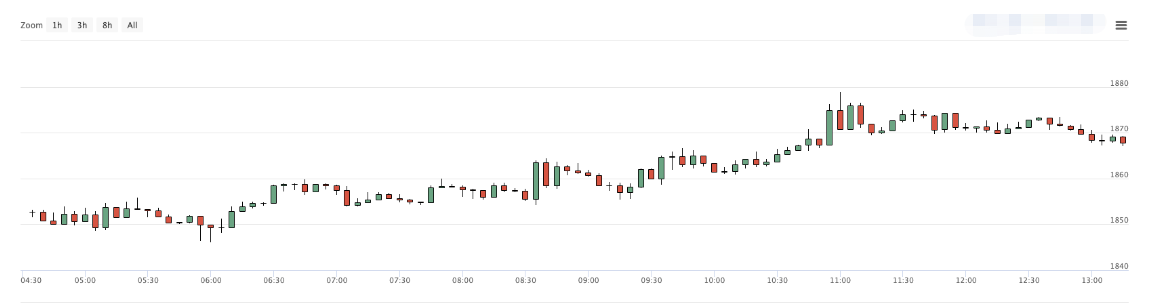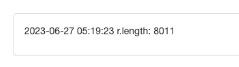Using the daily K-line data, we set it to retrieve 8000 bars (knowing that there is no market data available for 8000 days ago). This serves as a brute force test: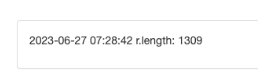Seeing that there are only 1309 daily K-lines, compare the data on the exchange charts: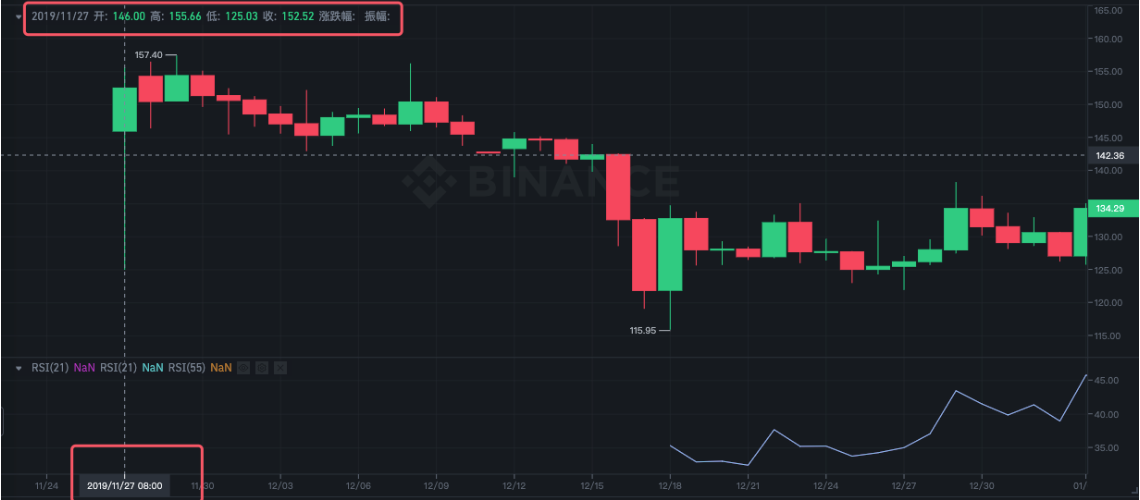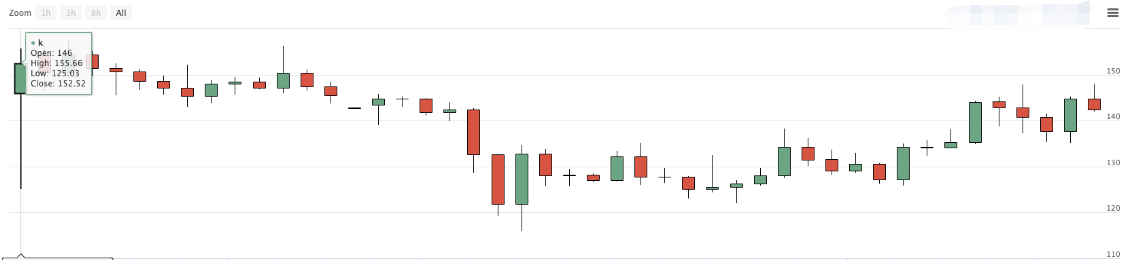You can see that the data also match.

## END

Template address: “JavaScript Version of Pagination Query K-Line Historical Data Template” Template address: “Plot Library”

The above template and strategy code are only for teaching and learning use, please optimize and modify according to the specific needs of the live trading.

Related

More